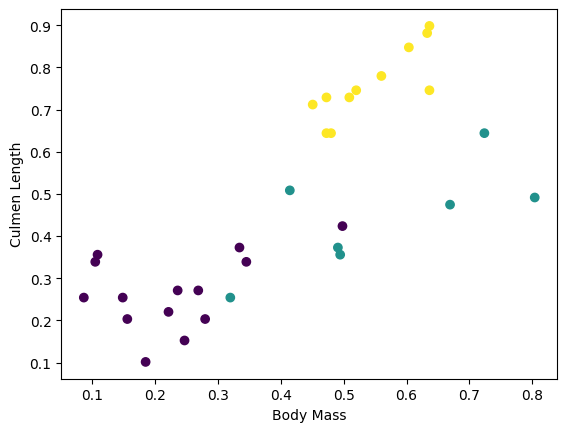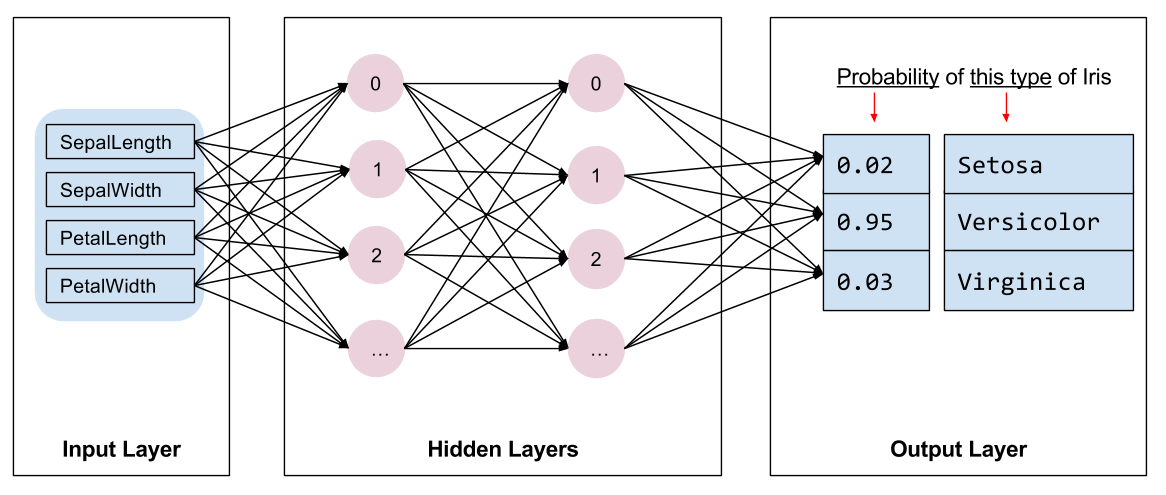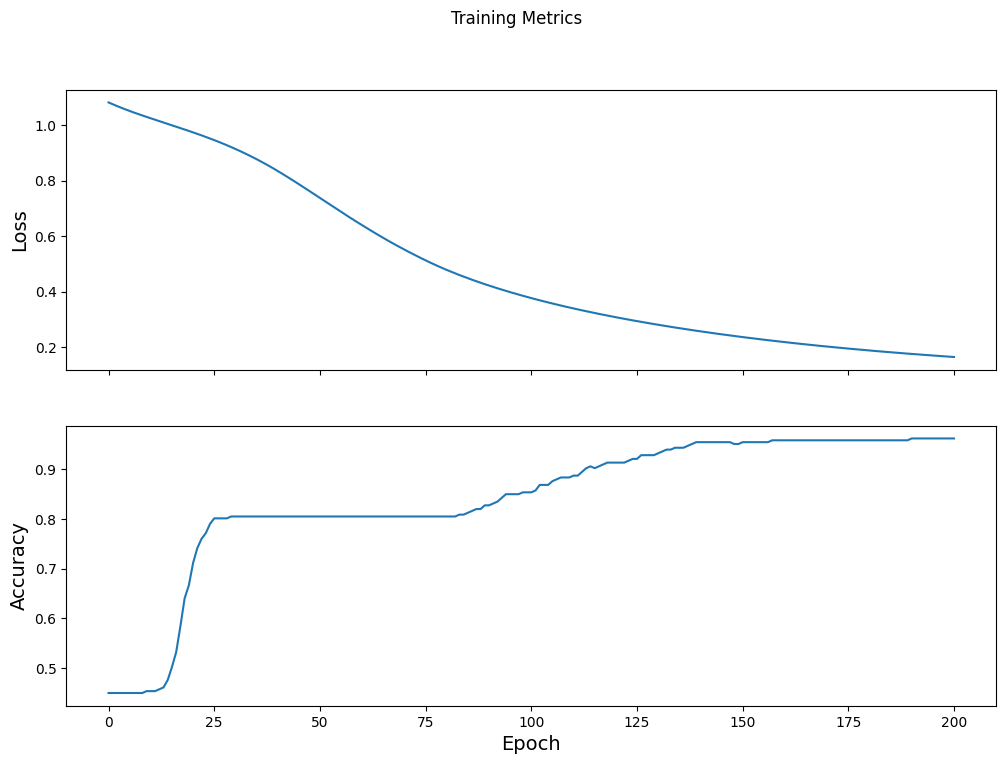# 自定义训练: 演示

1. 构建一个模型，
2. 用样例数据集对模型进行训练，以及
3. 利用该模型对未知数据进行预测。

## TensorFlow 编程

1. 数据集的导入与解析
2. 选择模型类型
3. 对模型进行训练
4. 评估模型效果
5. 使用训练过的模型进行预测

## 环境的搭建

### 配置导入

``````import os
import matplotlib.pyplot as plt
``````
``````import tensorflow as tf
``````
``````print("TensorFlow version: {}".format(tf.__version__))
print("Eager execution: {}".format(tf.executing_eagerly()))
``````
```TensorFlow version: 2.3.0
Eager execution: True

```

## 鸢尾花分类问题

• 山鸢尾
• 维吉尼亚鸢尾
• 变色鸢尾Figure 1. 山鸢尾 (by Radomil, CC BY-SA 3.0), 变色鸢尾, (by Dlanglois, CC BY-SA 3.0), and 维吉尼亚鸢尾 (by Frank Mayfield, CC BY-SA 2.0).

## 导入和解析训练数据集

### 下载数据集

``````train_dataset_url = "https://storage.googleapis.com/download.tensorflow.org/data/iris_training.csv"

train_dataset_fp = tf.keras.utils.get_file(fname=os.path.basename(train_dataset_url),
origin=train_dataset_url)

print("Local copy of the dataset file: {}".format(train_dataset_fp))
``````
```Downloading data from https://storage.googleapis.com/download.tensorflow.org/data/iris_training.csv
8192/2194 [================================================================================================================] - 0s 0us/step
Local copy of the dataset file: /home/kbuilder/.keras/datasets/iris_training.csv

```

### 检查数据

````head -n5 {train_dataset_fp}`
```
```120,4,setosa,versicolor,virginica
6.4,2.8,5.6,2.2,2
5.0,2.3,3.3,1.0,1
4.9,2.5,4.5,1.7,2
4.9,3.1,1.5,0.1,0

```

1. 第一行是表头，其中包含数据集信息：
• 共有 120 个样本。每个样本都有四个特征和一个标签名称，标签名称有三种可能。
• 后面的行是数据记录，每个样本各占一行，其中：
• 前四个字段是特征: 这四个字段代表的是样本的特点。在此数据集中，这些字段存储的是代表花卉测量值的浮点数。
• 最后一列是标签:即我们想要预测的值。对于此数据集，该值为 0、1 或 2 中的某个整数值（每个值分别对应一个花卉名称）。

``````# CSV文件中列的顺序
column_names = ['sepal_length', 'sepal_width', 'petal_length', 'petal_width', 'species']

feature_names = column_names[:-1]
label_name = column_names[-1]

print("Features: {}".format(feature_names))
print("Label: {}".format(label_name))
``````
```Features: ['sepal_length', 'sepal_width', 'petal_length', 'petal_width']
Label: species

```

• `0` : 山鸢尾
• `1` : 变色鸢尾
• `2` : 维吉尼亚鸢尾

``````class_names = ['Iris setosa', 'Iris versicolor', 'Iris virginica']
``````

### 创建一个 `tf.data.Dataset`

TensorFlow的 Dataset API 可处理在向模型加载数据时遇到的许多常见情况。这是一种高阶 API ，用于读取数据并将其转换为可供训练使用的格式。如需了解详情，请参阅数据集快速入门指南

``````batch_size = 32

train_dataset = tf.data.experimental.make_csv_dataset(
train_dataset_fp,
batch_size,
column_names=column_names,
label_name=label_name,
num_epochs=1)
``````

`make_csv_dataset` 返回一个`(features, label)` 对构建的 `tf.data.Dataset` ，其中 `features` 是一个字典: `{'feature_name': value}`

``````features, labels = next(iter(train_dataset))

print(features)
``````
```OrderedDict([('sepal_length', <tf.Tensor: shape=(32,), dtype=float32, numpy=
array([6.6, 5.8, 5. , 7.7, 4.6, 4.7, 5.5, 6.1, 6.5, 6.1, 5. , 6.4, 5.4,

6. , 5.5, 7.2, 5.9, 6.4, 5. , 5.2, 5. , 6.4, 6.2, 5.1, 6.4, 5.8,
5.1, 6.3, 6.5, 4.9, 7.4, 5.7], dtype=float32)>), ('sepal_width', <tf.Tensor: shape=(32,), dtype=float32, numpy=
array([2.9, 2.7, 3.4, 2.6, 3.1, 3.2, 2.4, 2.9, 3. , 2.6, 3.5, 3.1, 3.9,
3. , 2.4, 3.6, 3.2, 3.2, 3.2, 3.5, 2.3, 2.7, 3.4, 3.8, 2.8, 2.6,
2.5, 3.3, 3. , 3.1, 2.8, 3.8], dtype=float32)>), ('petal_length', <tf.Tensor: shape=(32,), dtype=float32, numpy=
array([4.6, 4.1, 1.5, 6.9, 1.5, 1.6, 3.8, 4.7, 5.5, 5.6, 1.6, 5.5, 1.7,
4.8, 3.7, 6.1, 4.8, 4.5, 1.2, 1.5, 3.3, 5.3, 5.4, 1.9, 5.6, 4. ,
3. , 6. , 5.8, 1.5, 6.1, 1.7], dtype=float32)>), ('petal_width', <tf.Tensor: shape=(32,), dtype=float32, numpy=
array([1.3, 1. , 0.2, 2.3, 0.2, 0.2, 1.1, 1.4, 1.8, 1.4, 0.6, 1.8, 0.4,
1.8, 1. , 2.5, 1.8, 1.5, 0.2, 0.2, 1. , 1.9, 2.3, 0.4, 2.2, 1.2,
1.1, 2.5, 2.2, 0.1, 1.9, 0.3], dtype=float32)>)])

```

``````plt.scatter(features['petal_length'],
features['sepal_length'],
c=labels,
cmap='viridis')

plt.xlabel("Petal length")
plt.ylabel("Sepal length")
plt.show()
````````````def pack_features_vector(features, labels):
"""将特征打包到一个数组中"""
features = tf.stack(list(features.values()), axis=1)
return features, labels
``````

``````train_dataset = train_dataset.map(pack_features_vector)
``````

`Dataset` 的特征元素被构成了形如 `(batch_size, num_features)` 的数组。我们来看看前几个样本:

``````features, labels = next(iter(train_dataset))

print(features[:5])
``````
```tf.Tensor(
[[5.  3.5 1.3 0.3]
[4.8 3.1 1.6 0.2]
[6.3 2.7 4.9 1.8]
[7.4 2.8 6.1 1.9]
[5.  3.2 1.2 0.2]], shape=(5, 4), dtype=float32)

```

## 选择模型类型

### 选择模型图 2. 包含特征、隐藏层和预测的神经网络

### 使用 Keras 创建模型

TensorFlow tf.keras API 是创建模型和层的首选方式。通过该 API，您可以轻松地构建模型并进行实验，而将所有部分连接在一起的复杂工作则由 Keras 处理。

tf.keras.Sequential 模型是层的线性堆叠。该模型的构造函数会采用一系列层实例；在本示例中，采用的是 2 个密集层（各自包含10个节点）,以及 1 个输出层（包含 3 个代表标签预测的节点。第一个层的 `input_shape` 参数对应该数据集中的特征数量，它是一项必需参数：

``````model = tf.keras.Sequential([
tf.keras.layers.Dense(10, activation=tf.nn.relu, input_shape=(4,)),  # 需要给出输入的形式
tf.keras.layers.Dense(10, activation=tf.nn.relu),
tf.keras.layers.Dense(3)
])
``````

### 使用模型

``````predictions = model(features)
predictions[:5]
``````
```<tf.Tensor: shape=(5, 3), dtype=float32, numpy=
array([[ 2.371686  , -3.2583737 ,  0.06788294],
[ 2.1781201 , -3.0004797 ,  0.07583394],
[ 1.4679078 , -2.8879187 , -0.13730617],
[ 1.60235   , -3.2915173 , -0.18439294],
[ 2.3404026 , -3.2052171 ,  0.06615102]], dtype=float32)>
```

``````tf.nn.softmax(predictions[:5])
``````
```<tf.Tensor: shape=(5, 3), dtype=float32, numpy=
array([[0.9062349 , 0.0032519 , 0.09051319],
[0.88667214, 0.00499719, 0.10833076],
[0.8239415 , 0.01057268, 0.16548584],
[0.85106575, 0.00637652, 0.14255764],
[0.90352327, 0.00352783, 0.09294892]], dtype=float32)>
```

``````print("Prediction: {}".format(tf.argmax(predictions, axis=1)))
print("    Labels: {}".format(labels))
``````
```Prediction: [0 0 0 0 0 0 0 0 0 0 0 0 0 0 0 0 0 0 0 0 0 0 0 0 0 0 0 0 0 0 0 0]
Labels: [0 0 2 2 0 2 0 1 0 0 1 1 0 1 0 0 2 2 1 1 0 2 2 0 0 2 1 0 0 0 2 2]

```

## 训练模型

### 定义损失和梯度函数

``````loss_object = tf.keras.losses.SparseCategoricalCrossentropy(from_logits=True)
``````
``````def loss(model, x, y):
y_ = model(x)

return loss_object(y_true=y, y_pred=y_)

l = loss(model, features, labels)
print("Loss test: {}".format(l))
``````
```Loss test: 1.6707830429077148

```

``````def grad(model, inputs, targets):
loss_value = loss(model, inputs, targets)
``````

### 创建优化器图 3. 优化算法在三维空间中随时间推移而变化的可视化效果。(来源: 斯坦福大学 CS231n 课程，MIT 许可证，Image credit: Alec Radford)

TensorFlow有许多可用于训练的优化算法。此模型使用的是 tf.train.GradientDescentOptimizer ， 它可以实现随机梯度下降法（SGD）。`learning_rate` 被用于设置每次迭代（向下行走）的步长。 这是一个 超参数 ，您通常需要调整此参数以获得更好的结果。

``````optimizer = tf.keras.optimizers.Adam(learning_rate=0.01)
``````

``````loss_value, grads = grad(model, features, labels)

print("Step: {}, Initial Loss: {}".format(optimizer.iterations.numpy(),
loss_value.numpy()))

print("Step: {},         Loss: {}".format(optimizer.iterations.numpy(),
loss(model, features, labels).numpy()))
``````
```Step: 0, Initial Loss: 1.6707830429077148
Step: 1,         Loss: 1.447718620300293

```

### 训练循环

1. 迭代每个周期。通过一次数据集即为一个周期。
2. 在一个周期中，遍历训练 `Dataset` 中的每个样本，并获取样本的特征`x`）和标签`y`）。
3. 根据样本的特征进行预测，并比较预测结果和标签。衡量预测结果的不准确性，并使用所得的值计算模型的损失和梯度。
4. 使用 `optimizer` 更新模型的变量。
5. 跟踪一些统计信息以进行可视化。
6. 对每个周期重复执行以上步骤。

`num_epochs` 变量是遍历数据集集合的次数。与直觉恰恰相反的是，训练模型的时间越长，并不能保证模型就越好。`num_epochs` 是一个可以调整的超参数。选择正确的次数通常需要一定的经验和实验基础。

``````## Note: 使用相同的模型变量重新运行此单元

# 保留结果用于绘制
train_loss_results = []
train_accuracy_results = []

num_epochs = 201

for epoch in range(num_epochs):
epoch_loss_avg = tf.keras.metrics.Mean()
epoch_accuracy = tf.keras.metrics.SparseCategoricalAccuracy()

# Training loop - using batches of 32
for x, y in train_dataset:
# 优化模型

# 追踪进度
epoch_loss_avg(loss_value)  # 添加当前的 batch loss
# 比较预测标签与真实标签
epoch_accuracy(y, model(x))

# 循环结束
train_loss_results.append(epoch_loss_avg.result())
train_accuracy_results.append(epoch_accuracy.result())

if epoch % 50 == 0:
print("Epoch {:03d}: Loss: {:.3f}, Accuracy: {:.3%}".format(epoch,
epoch_loss_avg.result(),
epoch_accuracy.result()))
``````
```Epoch 000: Loss: 1.470, Accuracy: 35.833%
Epoch 050: Loss: 0.112, Accuracy: 96.667%
Epoch 100: Loss: 0.055, Accuracy: 98.333%
Epoch 150: Loss: 0.065, Accuracy: 98.333%
Epoch 200: Loss: 0.053, Accuracy: 98.333%

```

### 可视化损失函数随时间推移而变化的情况

``````fig, axes = plt.subplots(2, sharex=True, figsize=(12, 8))
fig.suptitle('Training Metrics')

axes.set_ylabel("Loss", fontsize=14)
axes.plot(train_loss_results)

axes.set_ylabel("Accuracy", fontsize=14)
axes.set_xlabel("Epoch", fontsize=14)
axes.plot(train_accuracy_results)
plt.show()
``````## 评估模型的效果

5.93.04.31.511
6.93.15.42.122
5.13.31.70.500
6.0 3.4 4.5 1.6 12
5.52.54.01.311

### 建立测试数据集

``````test_url = "https://storage.googleapis.com/download.tensorflow.org/data/iris_test.csv"

test_fp = tf.keras.utils.get_file(fname=os.path.basename(test_url),
origin=test_url)
``````
```Downloading data from https://storage.googleapis.com/download.tensorflow.org/data/iris_test.csv
8192/573 [============================================================================================================================================================================================================================================================================================================================================================================================================================================] - 0s 0us/step

```
``````test_dataset = tf.data.experimental.make_csv_dataset(
test_fp,
batch_size,
column_names=column_names,
label_name='species',
num_epochs=1,
shuffle=False)

test_dataset = test_dataset.map(pack_features_vector)
``````

### 根据测试数据集评估模型

``````test_accuracy = tf.keras.metrics.Accuracy()

for (x, y) in test_dataset:
logits = model(x)
prediction = tf.argmax(logits, axis=1, output_type=tf.int32)
test_accuracy(prediction, y)

print("Test set accuracy: {:.3%}".format(test_accuracy.result()))
``````
```Test set accuracy: 96.667%

```

``````tf.stack([y,prediction],axis=1)
``````
```<tf.Tensor: shape=(30, 2), dtype=int32, numpy=
array([[1, 1],
[2, 2],
[0, 0],
[1, 1],
[1, 1],
[1, 1],
[0, 0],
[2, 2],
[1, 1],
[2, 2],
[2, 2],
[0, 0],
[2, 2],
[1, 1],
[1, 1],
[0, 0],
[1, 1],
[0, 0],
[0, 0],
[2, 2],
[0, 0],
[1, 1],
[2, 2],
[1, 2],
[1, 1],
[1, 1],
[0, 0],
[1, 1],
[2, 2],
[1, 1]], dtype=int32)>
```

## 使用经过训练的模型进行预测

• `0`: 山鸢尾
• `1`: 变色鸢尾
• `2`: 维吉尼亚鸢尾
``````predict_dataset = tf.convert_to_tensor([
[5.1, 3.3, 1.7, 0.5,],
[5.9, 3.0, 4.2, 1.5,],
[6.9, 3.1, 5.4, 2.1]
])

predictions = model(predict_dataset)

for i, logits in enumerate(predictions):
class_idx = tf.argmax(logits).numpy()
p = tf.nn.softmax(logits)[class_idx]
name = class_names[class_idx]
print("Example {} prediction: {} ({:4.1f}%)".format(i, name, 100*p))
``````
```Example 0 prediction: Iris setosa (99.9%)
Example 1 prediction: Iris versicolor (99.8%)
Example 2 prediction: Iris virginica (99.6%)

```